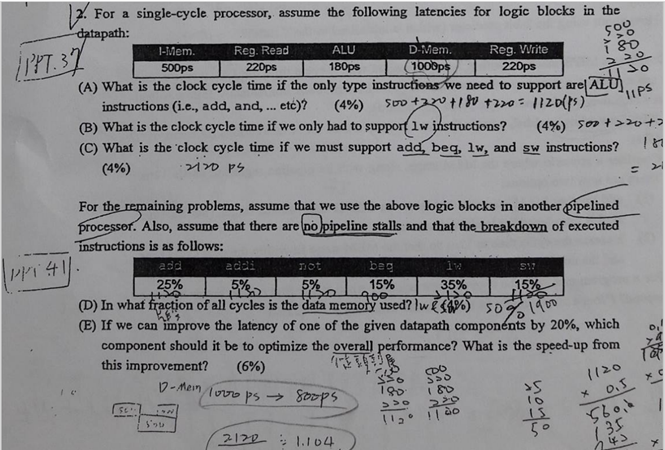2. For a single-cycle processor, assume the following latencies for logic blocks in the datapath: (A) What is the clock cycle time if the only type instructiens we need to support are|ALU $$\frac{200}{20}$$ instructions (i.e., add, and, ... etc)? (4\%) $$500+220+180+220=1120(p \mathrm{~s})$$ ALU $$11 \mathrm{P}^{\mathrm{s}}$$ (B) What is the clock cycle time if we only had to suppor 12 instructions? (C) What is the clock cycle time if we must support add, beq, $$\mathrm{lw}$$, and $$\mathrm{sw}$$ instructions? (4\%) $$\quad$$ iiro ps For the remaining problems, assume that we use the above logic blocks in another pipelined processer. Also, assume that there are no pipeline stalls and that the breakdown of executed instructions is as follows: (E) If we can improve the latency of one of the given datapath components by $$20 \%$$, which component should it be to optimize the overall performance? What is the speed-up from this improvement?

We have an Answer from Expert